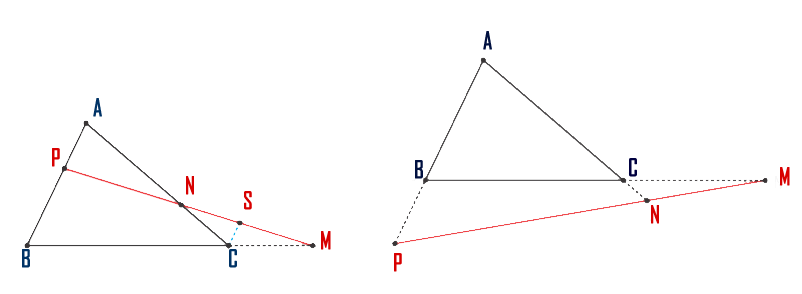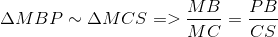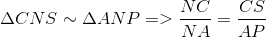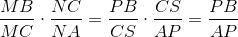# Menelaus' Theorem

## Famous theorems in 2D Geometry

Theorem. Whether the triangle ABC and the points M, N, P located on the lines BC, CA, AB respectively, different from A, B, C.
If the points M, N, P are collinear, then it has the relationship:Proof. We have 2 possible situations. As in bellow figure:We will make the proof only for the first situation. The second one is similar.

We draw CS || BA, S ∈ MP.

In the triangle MNP with CS || BP, according to the Fundamental Theorem of similarity, we have:And, again, inside the triangle CNS with CS || AP, we have:We multiply member by member the above relations and we have:and, finally:q.e.d.

Keywords: Menelaos, theorem, proof, geometry, school, education, Menelaus

### Forum

Here you can discuss about mathematic, about algebra, geometry, trigonometry.

It is not mandatory to be logged in on this forum but it is nice to have an account. You can ask about mathematics just with your name and your email.

This maths forum is one of the easiest forums to use it.

>> Go to Math Forum